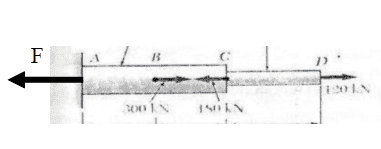# Force acting on steel rod (deformation)

in this problem , i could only understand that P3 = 120KN , i cant understand why P2= -60kN and why P1 = 240kN ? can someone explain ?

## The Attempt at a Solution

#### Attachments

Nidum
Gold Member
In the same way that you can do a shear force diagram for lateral loads on a beam you can do a tension force diagram for axial loads on a shaft .

Basically plot a graph of axial tension against position along shaft . Have a try .

In the same way that you can do a shear force diagram for lateral loads on a beam you can do a tension force diagram for axial loads on a shaft .

Basically plot a graph of axial tension against position along shaft . Have a try .
What do you mean? can you explain further?

Chestermiller
Mentor
If you do a force balance on the entire rod, what do you get for the reaction force on the left end?

Chestermiller
Mentor
how to do it ? i know only the P3 .... which is 120kN , how to do for P1 and P2 ?
You have 3 forces acting on the rod: 300 kN, 180 kN, and 120 kN. In order of the rod to be in static equilibrium, there must also be a force applied by the wall on the bar at location A. What does that force have to be?

You have 3 forces acting on the rod: 300 kN, 180 kN, and 120 kN. In order of the rod to be in static equilibrium, there must also be a force applied by the wall on the bar at location A. What does that force have to be?
what i found is this ? how to find the force applied by the wall on the bar at location A. ??
btw , i am not sure which 1 is P3 and which one is P2

#### Attachments

Chestermiller
Mentor
what i found is this ? how to find the force applied by the wall on the bar at location A. ??
btw , i am not sure which 1 is P3 and which one is P2
Forget about P1, P2, and P3 for now. Are you saying that you don't know how to do a 1 dimensional equilibrium force balance on a solid object?

•foo9008
Forget about P1, P2, and P3 for now. Are you saying that you don't know how to do a 1 dimensional equilibrium force balance on a solid object?
yes

Chestermiller
MentorF = 300 -180 + 120

•foo9008